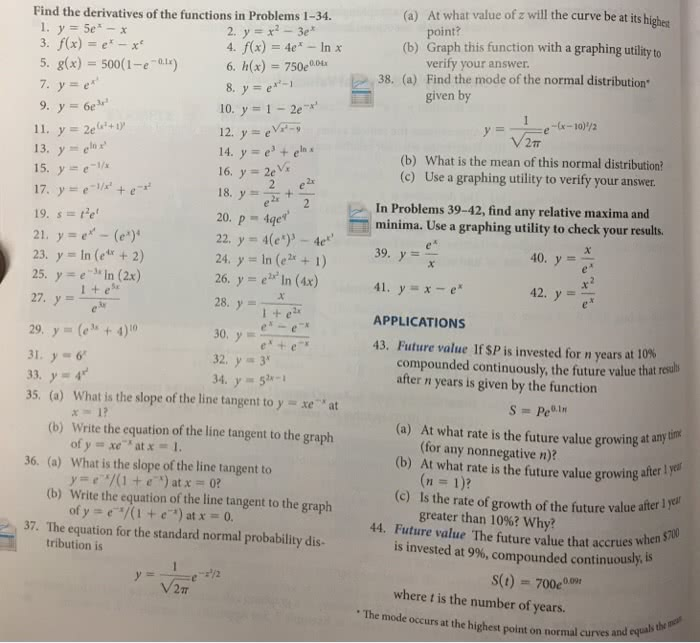1
0
watching
70
views13 Nov 2019

# Please answer for question #8, #10,#12,#44 With step and explation to solutions thank youAt what value of z will the curve be at its highest point? (a) (b) Graph this function with a graphing utlityto (a) Find the derivatives of the functions in Problems 1-34. 2, y=x2-3ex 4, f(x) = 4ex-In x 6. h(x) 750e004 . fx) -e 5Â·g(x) = 500(1-e-0.1x) 7, y=e 9, y=6e verity your answer Find the mode of the normal distribution. given by 38, 10. y = 1-2c" 2Ï (b) What is the mean of this normal distribution? (c) Use a graphing utility to verify your answer In x 15. y e 16, y = 2ev's /x+e In Problems 39-42, find any relative maxima and 19. ste 21, y=er_ (ex)' 23,y=ln (e4x + 2) 25, y=e-å·n (2x) 27, y= 20. p4e 22, y = 4(ex)"-4er' 24. y In (e 1) 26. yeIn (4x) 28, y = minima. Use a graphing utility to check your results. 40,y= 41, y=x-e APPLICATIONS 43. Future value If \$P is invested for n years at 10% 42, y=ex 29, y = (ex + 4)io 31 33, y=4" 35. (a) What is the slope of the line tangent to yxe "at compounded continuously, the future value that resul after n years is given by the function 32. y 3 (a) At what rate is the future value growing at any ih Write the equation of the line tangent to the graph ofy = xe-x at x = What is the slope of the line tangent to y=c"/(1 + e-x) atx = 0? (b) (for any nonnegative n)? (b) At what rate is the future value growing 1. after I yell 36. (a) (b) Write the equation of the line tangent to the graph (c) Is the rate of growth of the future value after 1 ofy = e-x/(1 + e-x) at x-0 greater than 10%? why? 37. The equation for the standard normal probability dis- 44. Future value The future value that accrues when is invested at 9%, compounded continuously. S(t) 700e tribution is where t is the number of years. The mode occurs at the highest point on normal curves thl and

For unlimited access to Homework Help, a Homework+ subscription is required.Elin HesselLv2
2 May 2019

## Unlock all answers

Get 1 free homework help answer.